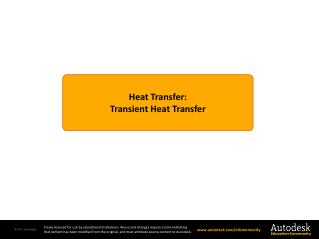DownloadDownload PresentationHeat Transfer : Transient Heat Transfer

# Heat Transfer : Transient Heat Transfer

Download Presentation## Heat Transfer : Transient Heat Transfer

- - - - - - - - - - - - - - - - - - - - - - - - - - - E N D - - - - - - - - - - - - - - - - - - - - - - - - - - -
##### Presentation Transcript

1. HeatTransfer: Transient Heat Transfer

2. Objectives Section 6 – Thermal Analysis Module5: Transient Heat Transfer Page 2 • Understand the basics of transient heat transfer. • Examine lumped mass systems. • Compare linear unsteady heat transfer with nonlinear unsteady heat transfer. • Study two examples: • Heat sink assembly temperature rise • Transient heat transfer through a rod

3. Transient Heat Transfer Section 6 – Thermal Analysis Module5: Transient Heat Transfer Page 3 • If systems can be approximated as lumped mass systems, transient heat transfer is easy to find. • Lumped mass systems rarely exist in reality, but particularly for large systems, this can prove a valuable approximation with little loss in accuracy. • For materials which are anisotropic rather than uniform, lumped mass systems may not be a good assumption. • The Biot number (Bi) is normally used to find if a lumped mass system would be applicable:

4. Simplifying Heat Transfer Analysis Section 6 – Thermal Analysis Module5: Transient Heat Transfer Page 4 • Lumped mass systems simplify transient simulation. The following equation is normally used: T(t) = Temperature after time t Tα = Ambient Temperature h = Heat transfer coefficient As = Surface Area V = Volume of lumped mass Cp = Specific heat of lumped mass

5. Temperature – Time Relationship Section 6 – Thermal Analysis Module5: Transient Heat Transfer Page 5 Where, The temperature of a lumped system approaches the temperature of the environment as time progresses. The above relationships enable us to determine the temperature T(t) of a body at time t, or alternatively, the time t required for the temperature to reach a specified value T(t).

6. Linear Unsteady Heat Transfer Section 6 – Thermal Analysis Module5: Transient Heat Transfer Page 6 • Temperature distribution throughout time is required. • The specific heat and the density of material is also required. • Computationally less expensive than nonlinear cases. • Very few cases for heat transfer in real life are linear unsteady because many thermophysical properties do change with temperature. The graph shows asymptotic rise of temperature with time.

7. Nonlinear Unsteady Heat Transfer Section 6 – Thermal Analysis Module5: Transient Heat Transfer Page 7 • Problems with unsteady temperature dependent conduction or involving radiation are nonlinear. • Exact solutions do not exist and an iterative scheme is used to solve such problems, as the number of unknowns is higher than the number of equations. • Because of nonlinearity, the time steps should be very small. • How often the nonlinear terms are updated can be controlled through programming.

8. Example: Heat Sink Assembly Temperature Rise Section 6 – Thermal Analysis Module5: Transient Heat Transfer Page 8 20° • A heat sink assembly example that was used in the earlier modules is used again here. For this module, we will be looking at the rise in temperature with time as the processor starts to dissipate heat from an initial ambient temperature of 20⁰C. • A video presentation is available with this module detailing the setting up and solving of transient heat transfer analysis for this example. B C Fins (Aluminium) Heat Spreader (Copper) 40 Watts Microprocessor (Silicon)

9. Additional Example: Transient Heat Transfer Through a Rod Section 6 – Thermal Analysis Module5: Transient Heat Transfer Page 9 • A solution for transient heat transfer of an insulated rod is available and can be compared to a simulation. • At the initial condition, the rod is hotter in the center and cooler at the ends, with a parabolic temperature distribution. Insulated rod is allowed to cool Problem Specification T=T0 T=T0 t =0 T(x, t=0) = f(x) and heat flux q=0 t → ∞ T0 T0 x =0 x =L Cooling Temperature Profile

10. Summary Section 6 – Thermal Analysis Module5: Transient Heat Transfer Page 10 • Transient heat transfer analysis occurs mostly during the starting/stopping of machinery, when the objective is to observe temperature variation with time; thus the additional variable of “time” has to be solved. • When solving analytically, the approximation of lumped mass systems can simplify the analysis. • For this approximation to be valid, the dimensionless Biot Number parameter is used.

11. Summary Section 6 – Thermal Analysis Module5: Transient Heat Transfer Page 11 • In the case of numerical analysis, the number of time steps will dictate the length of the analysis. • Therefore, provided that the gradient of temperature with time is low, larger time steps can be taken. • Just like steady state problems, transient heat transfer problems can be linear or nonlinear, with the latter adding another dimension of complexity into the mix.